### Home | Up One Level | Previous | Next

WALTER RITZ

#### Annales 271 (Oeuvres 422)

Between the new formulas and Lorentz’s theory, there is therefore no presently known contradiction in the domain of observed facts in electrodynamics. It is evident that from the viewpoint of mathematical elegance and simplicity, that the advantage will often be on the side of the theories of Lorentz; but, on the other hand, the latter can not always avoid the use of elementary forces. We have even seen that their use is indispensable in the theory of radiation. In these cases there will be equivalence. In fact, no deduction may be drawn from Lorentz’s equations before the demonstration has been done, which are often so complicated, that the movement of the Earth doesn’t noticeably influence the result; here, the advantage is on our side.

One of the most fruitful of Maxwell’s ideas has been the introduction of displacement currents, which form, along with conduction currents, a system of continuously closed currents to which Maxwell applied the known laws of electrodynamics (Neumann’s integral, etc.); it is thus that he arrived at his equations. The preceding developments show that this application constitutes a second hypothesis: indeed, we have shown that from our point of view and even from that of Lorentz, that these laws apply only to neutral currents, and this last point is, for (Oeuvres 423) Ampere’s laws for example, more important than the other; Maxwell’s hypothesis

comes back to the introduction of an elementary law for which all experimental basis is missing.

Our theory necessitates once again the distinction of open and closed currents. But if one considers not the mathematical formulas but the physical realities, one finds that the two orders of phenomena are quantitatively and qualitatively so different that the practical utility of their synthesis is not perhaps as great as it seemed at first glance.

The formula for the action of a mobile electrified point on another, to which Lorentz theory leads, is very analogous as Schwarzschild(1) has remarked, to that of Clausius(2), which contains absolute speeds as well. The latter arrived at this by admitting, among other things, that there is no action between a galvanic current and an electric charge at rest. This is very obviously true for neutral currents, as we have seen, but could be absolutely false in other cases. This hypothesis leads, according to Clausius as well as Lorentz, to the introduction of absolute movement.

We have seen that, in the expression of the elementary force, a factor k remains indeterminate. This calls to mind the analogous result of Helmholtz, and likewise formula (25), which is applicable to elements of neutral current, is identical to those of Helmholtz(3) and reduces to that of Ampere for k = -1, and to that of F. Neumann, Maxwell, and Lorentz for k = +1. The couples that are added in Helmholtz’s theory are lacking, however, and this point is essential. Moreover, we now know that only in the case where there is no radiation does the energy remain constant; the relations that the energy equation involves between the open current’s inductive actions      ___________________________________________________________________________________________

(1) Göttinger Nachrichten, 1903.

(2) Journal für Math. (Crelle), v.82, 1877, p.85.

(3) Wissenschaft. Abhandl., v.I, p.688, Leipzig, 1882.

and their electrodynamic actions can consequently cease to be satisfied. This is indeed what happens. For induction phenomena inside bodies at rest, the equations of Maxwell-Lorentz and those of Helmholtz(4) become identical if k = 0, as the latter has remarked. In this (Oeuvres 424) case, only the resistance, the electrostatic force and the accelerations play a role. Lorentz’s formulas are then identical to ours, which also correspond to k = 0. As to the consequences relative to stability, requiring k ≥ 0, they can only apply to the value of k that figures in induction phenomena (it is enough, to see it, to suppose the currents effectively nil). Our formulas therefore always satisfy, and our parameter k stays entirely undetermined.

It is interesting to notice that, in bodies at rest, induction phenomena in a closed circuit come only from the finite propagation speed in our theory. Indeed if we refer to the developments of section 3, we see that as far as the terms of the second order are concerned only this finite speed introduces accelerations, and it is these latter [ones] which determine the induction phenomena, for the term of the second order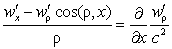which does not come from these developments gives a nil electromotive force for a closed circuit. We know that, in the hypothesis for actions at a distance, the induction phenomena have been deduced from electrostatic and electrodynamic forces, starting from the energy equation; in our theory, they are deduced from propagation.

We could determine the k factor by adopting the theory of metals, proposed by Riecke and Drude, ___________________________________________________________________________________________

(4) Loc. cit., p.573, equation (3b).

according to which the speed of electrons in their irregular molecular movement would be much greater than that of the positive ions, and which amounts to tens or hundreds kilometers per second. Let

dt' be an element of volume of the metal;

E'dt' be the total charge of the electrons in this element;

v' be their mean speed.

The body not being electrified, the action of dt' on a charge e situated in xyz will be by symmetry, considering the irregularities of the movements, parallel to r, and equation (VI) gives for this force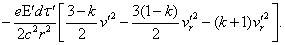Now we have, on the average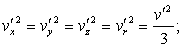(Oeuvres 425) the force is therefore

(54)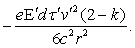Everything happens as if the body carried an electric charge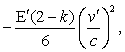proportional, like v'², to the absolute temperature, and quite considerable; since this cannot be, it must be that k is equal to two. It is true that, if such an action existed, it would no longer be barely possible to demonstrate, nor doubtless even to admit, Boltzmann’s theory relative to the distribution of energy between independent coordinates, on which is founded the hypothesis of large values of v'. If the molecular speeds of positive and negative ions are, on the contrary, essentially equal, force (54) would cease to exist, except perhaps for electro-thermal phenomena.

We have seen that the new theory represents

hertzian oscillations well. The fictitious particles are thus periodically distributed in time and space. This distribution in turn provokes the oscillations of other ions or systems of ions; the combination of these actions by interference, that is to say, by simple superposition, then gives rise to the diverse phenomena of reflection, refraction, etc.

When one can consider the speeds and the amplitude of the accelerations of ions as being infinitely small, the concordance between Lorentz’s formulas, and mine demonstrated for hertzian oscillations, continues to exist no matter what the frequency is. With this restriction, both would represent optical phenomena. But when the speeds intervene, for example in Fizeau’s experiment on the entrainment of waves, the agreement ceases; our formulas demand, as do those of Hertz, total entrainment. I said in the Introduction that one could eliminate the difficulty by admitting a certain reaction on the part of electric charges caused by the movement of the [fictitious] particles.

Let us remark in conclusion, that our law of propagation effortlessly reconciles the phenomenon of aberration and Michelson’s experiment, which seemed contradictory in the theory of ether. Indeed, in the second experiment, the light sources being (Oeuvres 426) drawn along in the Earth’s movement, the speed of their light in relation to the latter has a different value than that of the speed of light from the stars, which is independent of the Earth’s movement; the emanative theory of light which gives such a simple explanation of aberration will subsist in its essential features.